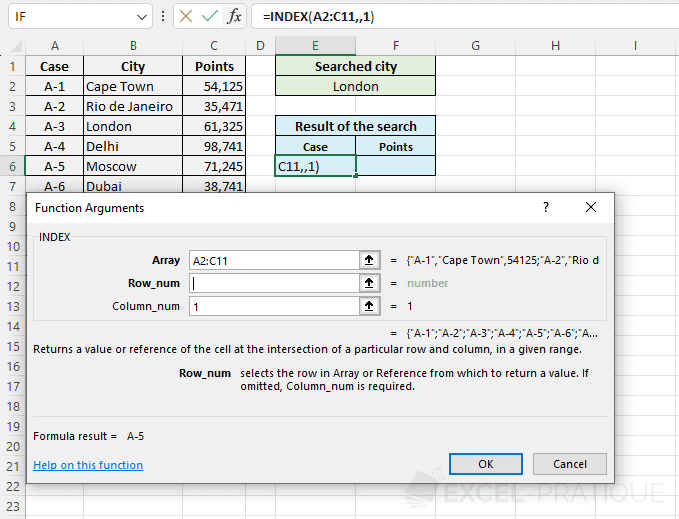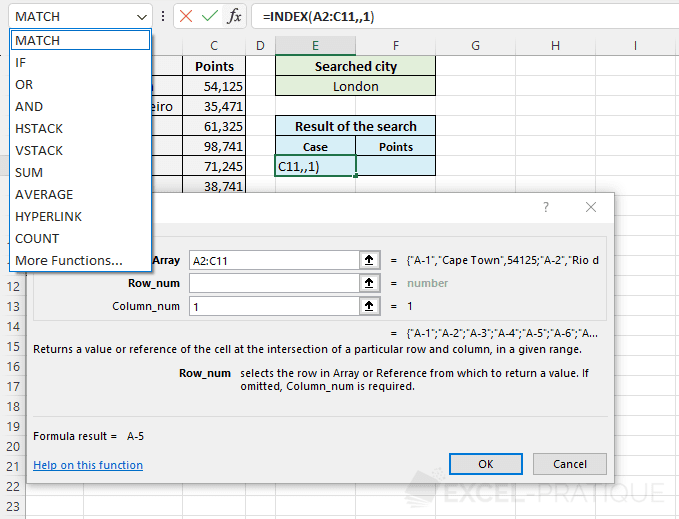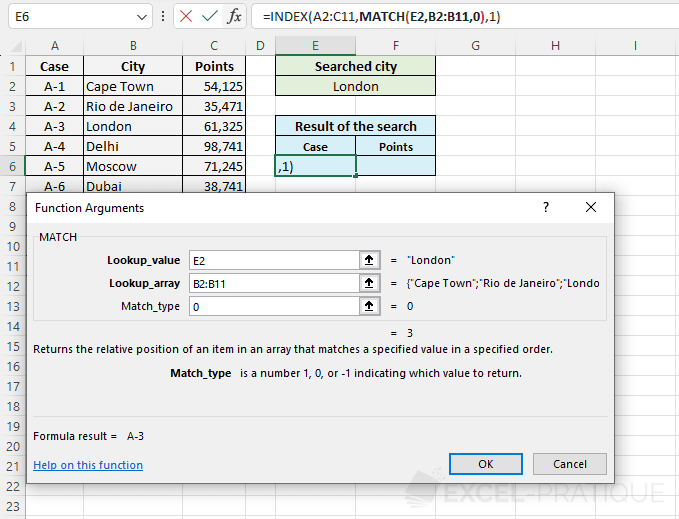# Excel functions: INDEX + MATCH

The INDEX function used together with the MATCH function allows us to search for a value in an array.

Let's try an example:

We want to be able to find a person's age and/or city based on his or her first name (this could also be done based on age or city).

Here is the formula:

`=INDEX(B2:D10,MATCH(G4,B2:B10,0),H6)`

• B2:D10: Lookup array
• G4: Lookup value (in this case, the first name)
• B2:B10: cell range within which G4 is to be found (in this case, the first names)
• 0: search for exact value (1: largest value / -1: smallest value)
• H6: number of the column that contains the values to be displayed (in this case, the cities)If we edit H6, we will get:Note:

In this example, we have used the following syntax:

`=INDEX(array,MATCH(lookup_value,array,0),column_num)`

We could also have used the syntax below (in this version, the result returned by the MATCH function will be the column number instead of the row number):

`=INDEX(array,row_num,MATCH(lookup_value,array,0))`